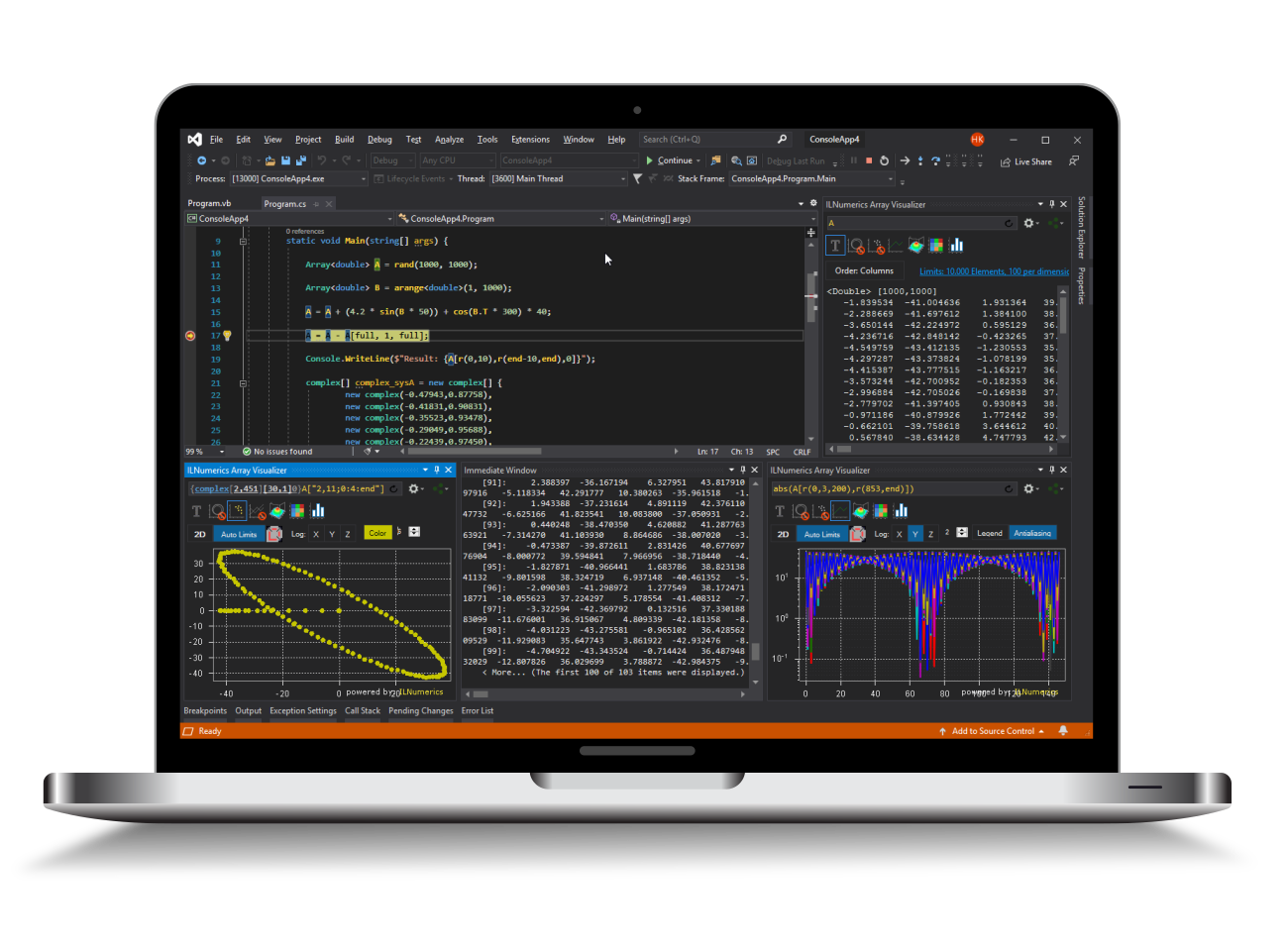Industrial Data Science
in C# and .NET:
Simple. Fast. Reliable.ILNumerics - Technical Computing

Modern High Performance Tools for Technical

Computing and Visualization in Industry and Science

tgt

# Linear Regression in .NET (C# and Visual Basic)

A simple linear regression model aims to model a set of measurement data with a line. The slope and the offset of the line are directly computed from the measurement data. Here comes one variant, which utilizes ILMath.pinv in order to compute the slope and ILMath.mean to compute the offset.

Often, the solution of least squares problems is found by defining the problems in terms of a design matrix. This includes the measurement data and (optionally) powers of it (for polynomial regression).